Courses
Courses for Kids
Free study material
Free LIVE classes
More

# Heptagon - Important Concepts with Examples for JEECan you spot any seven-sided objects when you look around? These objects can range from old coins, vases, paperweights, erasers, pencils, and so on. These objects are heptagonal in shape. A heptagon is a geometric shape with seven sides. In the following sections, we will discuss the definition of a heptagon, the shape and types of heptagons, the properties of heptagons, and some real-life examples of heptagon shapes.

So, let’s first learn the definition of a heptagon.

## What is a Heptagon?

A heptagon can be defined as a polygon that has seven sides, seven angles, and seven edges. The sum of all the angles of a heptagon is 900°. Fourteen diagonals can be drawn in a heptagon. Sometimes heptagon is also known as septagon. The word ‘Heptagon’ is a combination of two Greek words ‘heptá’ which means seven and ‘gōnía’ which means angle.

## What does a Heptagon Shape Look Like?Heptagon Shape

As we can see in the above image, all the seven sides meet with each other end to end and form the heptagon shape.

## Types of Heptagon Shapes

Based on the sides and angles, there are different kinds of heptagon shapes.

Based on the side length of the heptagons, heptagons can be categorised into the following two types.

• Regular Heptagon

All sides of a regular heptagon are equal in length and all the angles are equal in measure. The sum of all the interior angles of the regular heptagon is 900°. So, each of the interior angles measures 900°/7, i.e., 128.57°.Shape of a Regular Heptagon

• Irregular Heptagon

All sides and angles of an irregular heptagon have different measures. Each of the interior angles of an irregular heptagon is different in measure but the sum of the interior angles is 900°.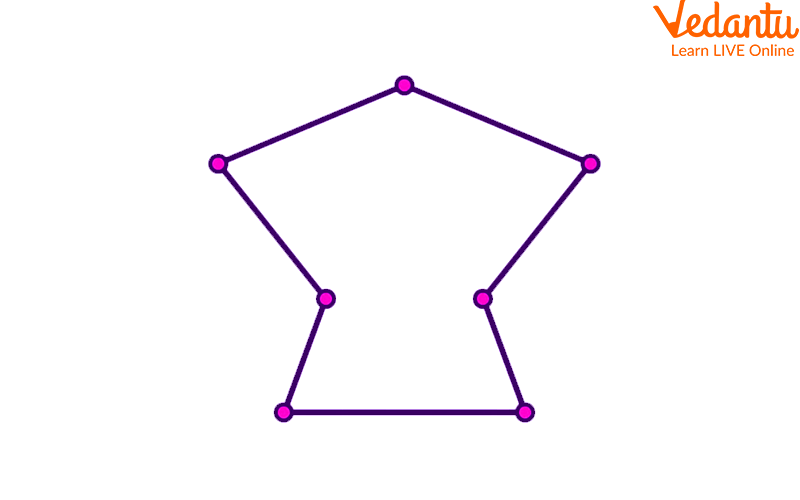Irregular Heptagon

Based on the angle measures, the heptagon can be classified into the following two types.

• Convex Heptagon

In a convex heptagon, the value of the interior angles is less than 180°. So, no diagonals of the convex heptagon can lie outside the polygon.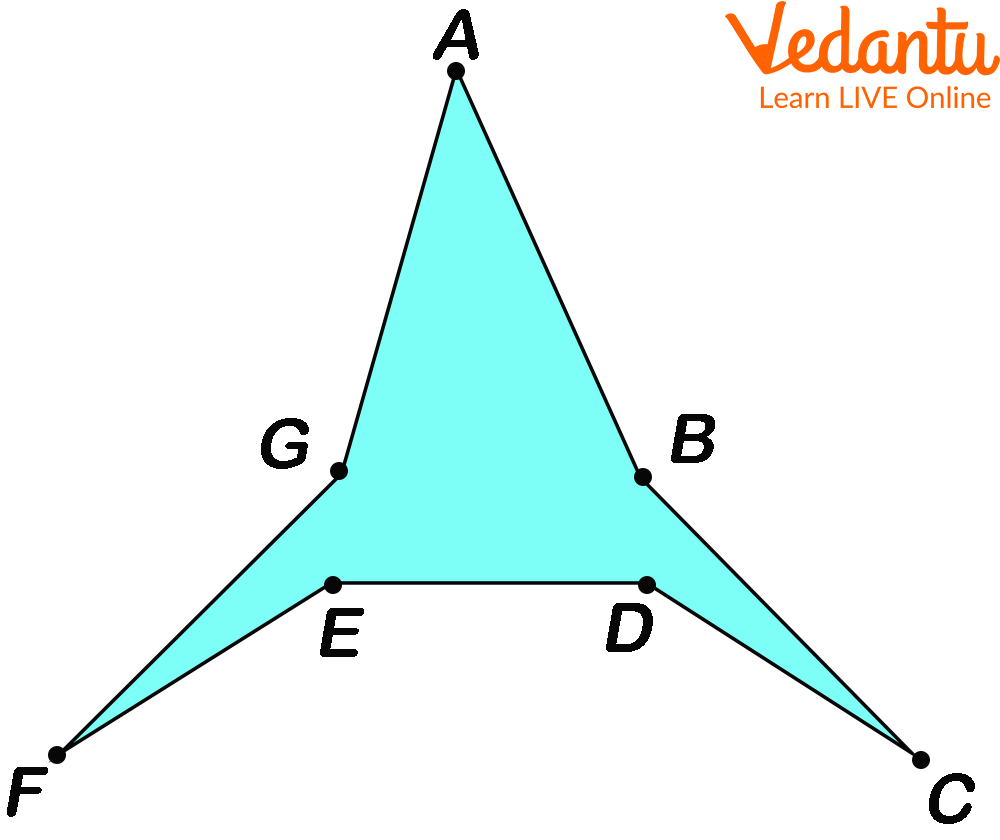Convex Heptagon

• Concave Heptagon

In a concave heptagon, at least one of the interior angles is greater than 180° and at least one of the diagonals lies outside the polygon.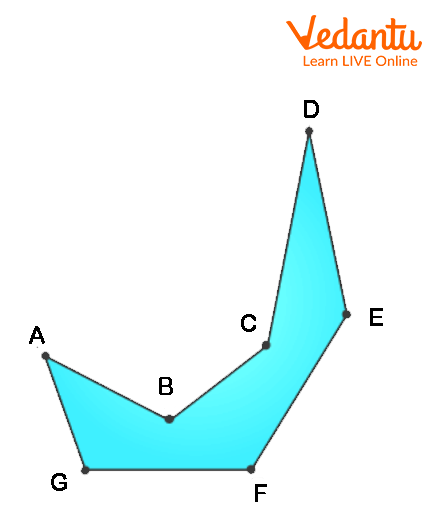Concave Heptagon

## Heptagon Properties

The key properties of a heptagon help us to identify a heptagon shape easily. The properties are as follows.

• A heptagon has seven angles, seven sides, and seven vertices.

• The sum of all the interior angles in a heptagon is equal to 900 degrees.

• The sum of all the exterior angles of a heptagon is equal to 360 degrees.

• In a regular heptagon, the value of each of the interior angles is approximately 128.57 degrees.

• The value of the central angle of a regular heptagon is approximately 51.43 degrees.

• Fourteen diagonals can be drawn in a heptagon.

• Regular heptagons are also known as convex heptagons because all of their interior angles measure less than 180 degrees.

• Five triangles can be formed in a heptagon.

## A Few Real-Life Examples of Heptagons

We have created a list of some real-life examples of heptagons. Check out the list and see whether you can find any of these objects around you and try to see if you have got your own to add to this list.

 Examples Shapes Storage Box: Medicine boxes or storage containers mostly have 7 small plastic cases attached to each other. So, a storage box is a proper example of the heptagon shape used in daily life.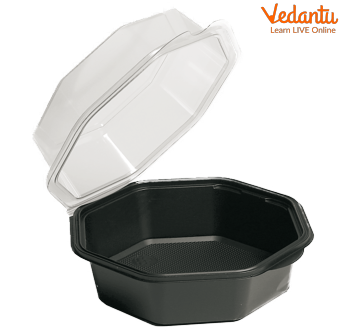Coins: Some of the old coins are heptagonal in shape. This unique structure of the coins helps us to easily differentiate these coins from the others.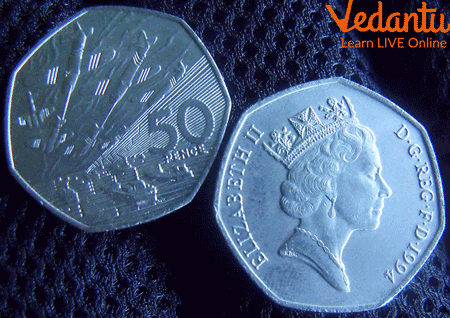Cactus: One of the perfect examples of the heptagonal shape is a cactus that exists in nature. If you look at it from the top, you can easily observe the stems of the cactus form the seven sides of the heptagon.Paper Boat: A classic example of an irregular heptagon is a paper boat. If you look at the outer boundary of a paper boat, you can observe that it has seven sides, seven edges, and seven angles, and the sides and the angles are of different measures.Arrowhead: An arrowhead is another example of an irregular heptagon. If you draw an arrowhead on a piece of paper, you will see that it has seven unequal sides and seven unequal angles.Candle holders: Nowadays, some candle holders are manufactured in heptagon shape.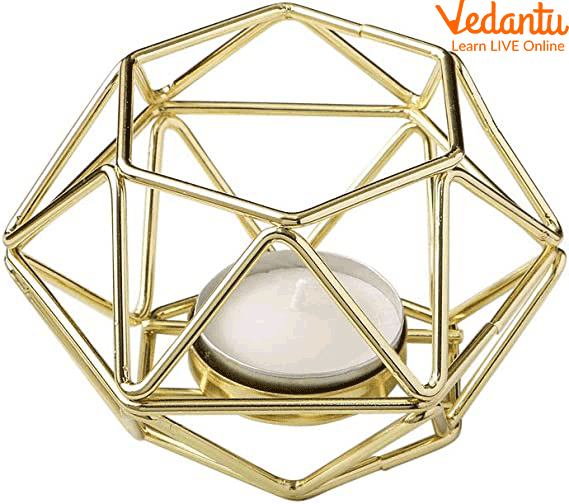Green House: Some of the small and compact greenhouses that are usually installed in the backyards are of heptagonal shape.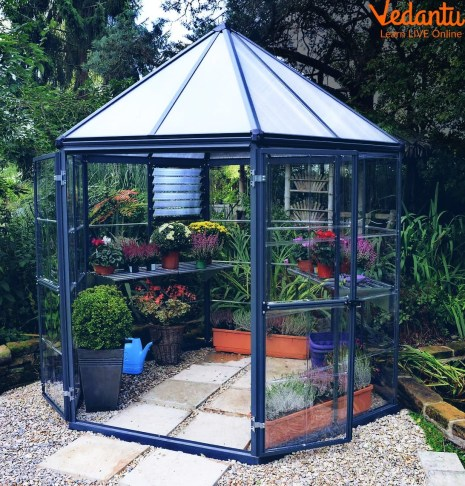Heptagonal Star: For the purpose of decoration, heptagonal stars are used. They are formed by joining the diagonals of a heptagonal geometric figure.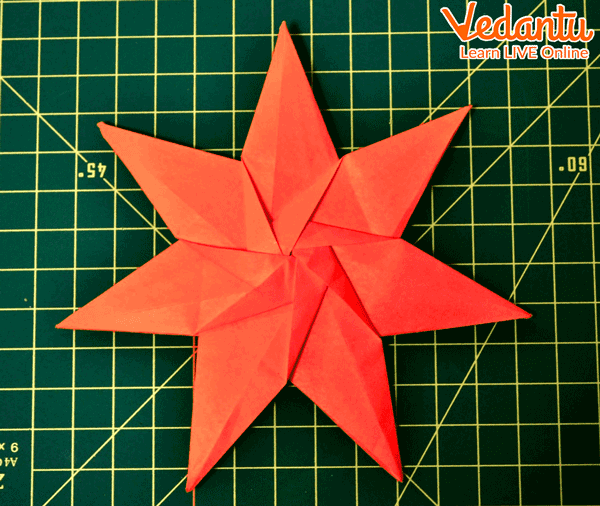Now, we hope that it is clear to you what the shape of a heptagon looks like and here we have learned about different types of heptagons and their properties. We have also provided some real-life examples of the heptagon. This information will be really helpful while studying geometry.

Last updated date: 01st Oct 2023
Total views: 131.4k
Views today: 1.31k

## FAQs on Heptagon - Important Concepts with Examples for JEE

1. What is the difference between regular and irregular heptagon?

In a regular heptagon, all the angles and sides are equal while an irregular heptagon has all sides and angles of different measures.

2. How many diagonals does a heptagon have?

A heptagon has 14 diagonals.